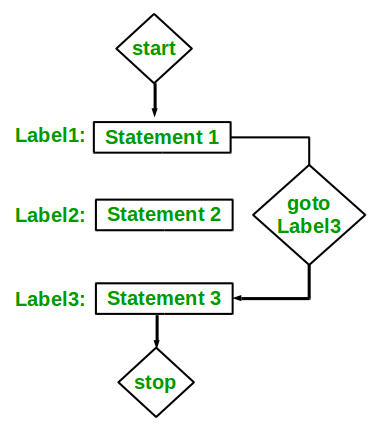# goto statement in C/C++

• Difficulty Level : Easy
• Last Updated : 26 Aug, 2019

The goto statement is a jump statement which is sometimes also referred to as unconditional jump statement. The goto statement can be used to jump from anywhere to anywhere within a function.
Syntax:

```Syntax1      |   Syntax2
----------------------------
goto label;  |    label:
.            |    .
.            |    .
.            |    .
label:       |    goto label;
```

In the above syntax, the first line tells the compiler to go to or jump to the statement marked as a label. Here label is a user-defined identifier which indicates the target statement. The statement immediately followed after ‘label:’ is the destination statement. The ‘label:’ can also appear before the ‘goto label;’ statement in the above syntax.Below are some examples on how to use goto statement:
Examples:

Take a step-up from those "Hello World" programs. Learn to implement data structures like Heap, Stacks, Linked List and many more! Check out our Data Structures in C course to start learning today.
• Type 1: In this case, we will see a situation similar to as shown in Syntax1 above. Suppose we need to write a program where we need to check if a number is even or not and print accordingly using the goto statement. Below program explains how to do this:

## C

 `// C program to check if a number is``// even or not using goto statement``#include `` ` `// function to check even or not``void` `checkEvenOrNot(``int` `num)``{``    ``if` `(num % 2 == 0)``        ``// jump to even``        ``goto` `even; ``    ``else``        ``// jump to odd``        ``goto` `odd; `` ` `even:``    ``printf``(``"%d is even"``, num);``    ``// return if even``    ``return``; ``odd:``    ``printf``(``"%d is odd"``, num);``}`` ` `int` `main() {``    ``int` `num = 26;``    ``checkEvenOrNot(num);``    ``return` `0;``}`

## C++

 `// C++ program to check if a number is``// even or not using goto statement``#include ``using` `namespace` `std;`` ` `// function to check even or not``void` `checkEvenOrNot(``int` `num)``{``    ``if` `(num % 2 == 0)``    ``// jump to even``        ``goto` `even; ``    ``else``    ``// jump to odd``        ``goto` `odd; `` ` `even:``    ``cout << num << ``" is even"``;``    ``// return if even``    ``return``; ``odd:``    ``cout << num << ``" is odd"``;``}`` ` `// Driver program to test above function``int` `main()``{``    ``int` `num = 26;``    ``checkEvenOrNot(num);``    ``return` `0;``}`
Output:

```26 is even
```
• Type 2:: In this case, we will see a situation similar to as shown in Syntax1 above. Suppose we need to write a program which prints numbers from 1 to 10 using the goto statement. Below program explains how to do this.

## C

 `// C program to print numbers``// from 1 to 10 using goto statement``#include `` ` `// function to print numbers from 1 to 10``void` `printNumbers()``{``    ``int` `n = 1;``label:``    ``printf``(``"%d "``,n);``    ``n++;``    ``if` `(n <= 10)``        ``goto` `label;``}`` ` `// Driver program to test above function``int` `main() {``    ``printNumbers();``    ``return` `0;``}`

## C++

 `// C++ program to print numbers``// from 1 to 10 using goto statement``#include ``using` `namespace` `std;`` ` `// function to print numbers from 1 to 10``void` `printNumbers()``{``    ``int` `n = 1;``label:``    ``cout << n << ``" "``;``    ``n++;``    ``if` `(n <= 10)``        ``goto` `label;``}`` ` `// Driver program to test above function``int` `main()``{``    ``printNumbers();``    ``return` `0;``}`
Output:
```1 2 3 4 5 6 7 8 9 10
```